# Problem: A hand pushes three identical bricks as shown. The bricks are moving to the left and speeding up. System A consists of two bricks stacked together. System B consists of a single brick. System C consists of all three bricks. There is friction between the bricks and the table.a) Draw and label separate free-body diagrams for systems A and B.b) The vector representing the acceleration of system A is shown below. Draw the acceleration vectors for systems B and C using the same scale (shown incorrectly in figure). Explain.c) The vector representing the net force on system A is shown below. Draw the net force vectors for systems B and C using the same scale. Explain.d) The vector representing the frictional force on system A is shown below. Draw the remaining force vectors using the same scale. Explain how you knew to draw the force vectors as you did.

###### FREE Expert Solution

a) The free-body diagram for system A: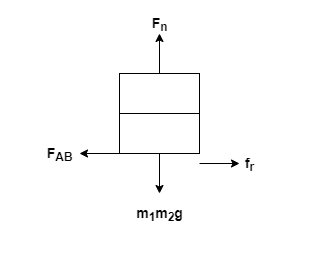Fn = Normal force, fr = friction force, FAB = force of B on A, and m1m2g = weight of m1 and m2

82% (194 ratings)###### Problem Details

A hand pushes three identical bricks as shown. The bricks are moving to the left and speeding up. System A consists of two bricks stacked together. System B consists of a single brick. System C consists of all three bricks. There is friction between the bricks and the table.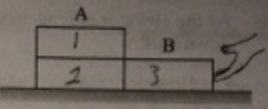a) Draw and label separate free-body diagrams for systems A and B.

b) The vector representing the acceleration of system A is shown below. Draw the acceleration vectors for systems B and C using the same scale (shown incorrectly in figure). Explain.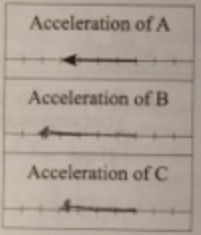c) The vector representing the net force on system A is shown below. Draw the net force vectors for systems B and C using the same scale. Explain.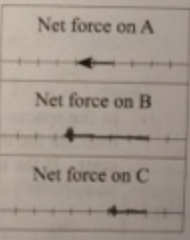d) The vector representing the frictional force on system A is shown below. Draw the remaining force vectors using the same scale.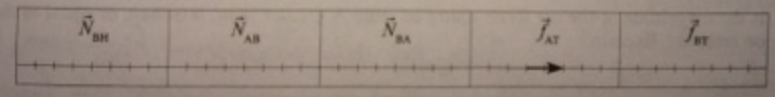Explain how you knew to draw the force vectors as you did.